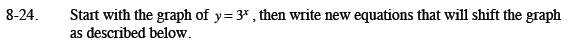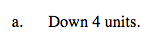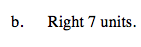Home > CCA2 > Chapter 8 > Lesson 8.1.1 > Problem8-24

8-24.
1. Start with the graph of y = 3x, then write new equations that will shift the graph as described below. Homework Help ✎

1. Down 4 units.

2. Right 7 units.$y = 3^{x - 7}$